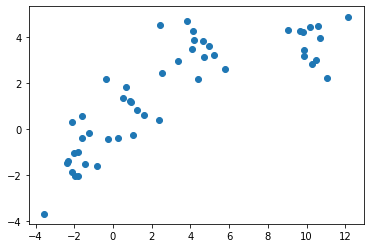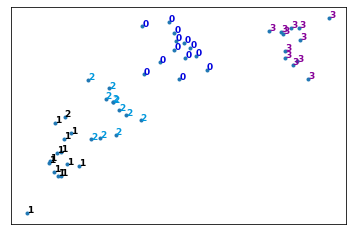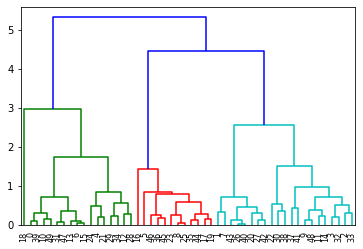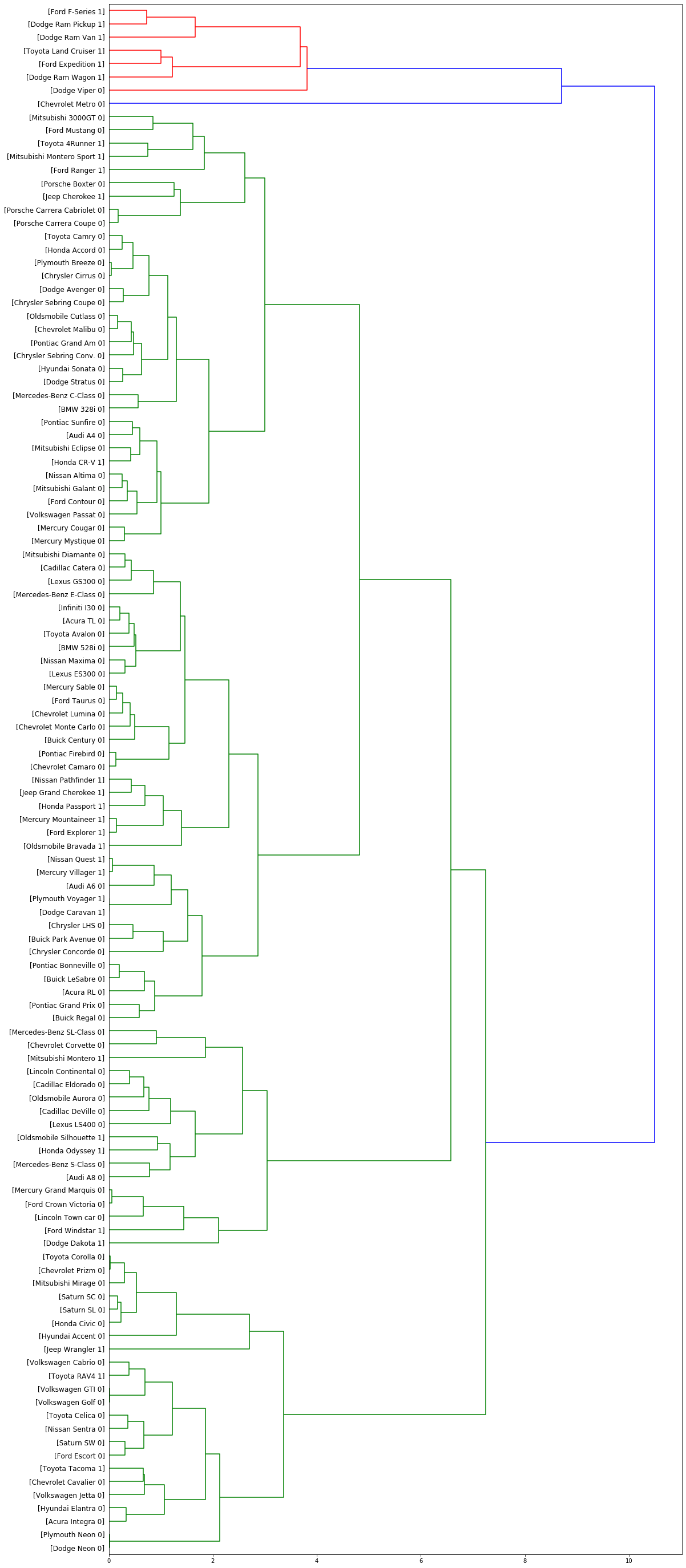0%

Welcome to Lab of Hierarchical Clustering with Python using Scipy and Scikit-learn package.

# Hierarchical Clustering - Agglomerative

We will be looking at a clustering technique, which is Agglomerative Hierarchical Clustering. Remember that agglomerative is the bottom up approach.

In this lab, we will be looking at Agglomerative clustering, which is more popular than Divisive clustering.

NOTE: You can also try using Average Linkage wherever Complete Linkage would be used to see the difference!

In :
```import numpy as np
import pandas as pd
from scipy import ndimage
from scipy.cluster import hierarchy
from scipy.spatial import distance_matrix
from matplotlib import pyplot as plt
from sklearn import manifold, datasets
from sklearn.cluster import AgglomerativeClustering
from sklearn.datasets.samples_generator import make_blobs
%matplotlib inline
```

### Generating Random Data

We will be generating a set of data using the make_blobs class.

Input these parameters into make_blobs:

• n_samples: The total number of points equally divided among clusters.
• Choose a number from 10-1500
• centers: The number of centers to generate, or the fixed center locations.
• Choose arrays of x,y coordinates for generating the centers. Have 1-10 centers (ex. centers=[[1,1], [2,5]])
• cluster_std: The standard deviation of the clusters. The larger the number, the further apart the clusters
• Choose a number between 0.5-1.5
</ul>
Save the result to X1 and y1.

In :
```X1, y1 = make_blobs(n_samples=50, centers=[[4,4], [-2, -1], [1, 1], [10,4]], cluster_std=0.9)
```

Plot the scatter plot of the randomly generated data

In :
```plt.scatter(X1[:, 0], X1[:, 1], marker='o')
```
Out:
`<matplotlib.collections.PathCollection at 0x7fae5b5b0518>`### Agglomerative Clustering

We will start by clustering the random data points we just created.

The Agglomerative Clustering class will require two inputs:

• n_clusters: The number of clusters to form as well as the number of centroids to generate.
• Value will be: 4
• linkage: Which linkage criterion to use. The linkage criterion determines which distance to use between sets of observation. The algorithm will merge the pairs of cluster that minimize this criterion.
• Value will be: 'complete'
• Note: It is recommended you try everything with 'average' as well
</ul>
Save the result to a variable called agglom

In :
```agglom = AgglomerativeClustering(n_clusters = 4, linkage = 'average')
```

Fit the model with X2 and y2 from the generated data above.

In :
```agglom.fit(X1,y1)
```
Out:
```AgglomerativeClustering(affinity='euclidean', compute_full_tree='auto',
n_clusters=4, pooling_func='deprecated')```

Run the following code to show the clustering!
Remember to read the code and comments to gain more understanding on how the plotting works.

In :
```# Create a figure of size 6 inches by 4 inches.
plt.figure(figsize=(6,4))

# These two lines of code are used to scale the data points down,
# Or else the data points will be scattered very far apart.

# Create a minimum and maximum range of X1.
x_min, x_max = np.min(X1, axis=0), np.max(X1, axis=0)

# Get the average distance for X1.
X1 = (X1 - x_min) / (x_max - x_min)

# This loop displays all of the datapoints.
for i in range(X1.shape):
# Replace the data points with their respective cluster value
# (ex. 0) and is color coded with a colormap (plt.cm.spectral)
plt.text(X1[i, 0], X1[i, 1], str(y1[i]),
color=plt.cm.nipy_spectral(agglom.labels_[i] / 10.),
fontdict={'weight': 'bold', 'size': 9})

# Remove the x ticks, y ticks, x and y axis
plt.xticks([])
plt.yticks([])
#plt.axis('off')

# Display the plot of the original data before clustering
plt.scatter(X1[:, 0], X1[:, 1], marker='.')
# Display the plot
plt.show()
```### Dendrogram Associated for the Agglomerative Hierarchical Clustering

Remember that a distance matrix contains the distance from each point to every other point of a dataset .
Use the function distance_matrix, which requires two inputs. Use the Feature Matrix, X2 as both inputs and save the distance matrix to a variable called dist_matrix

Remember that the distance values are symmetric, with a diagonal of 0's. This is one way of making sure your matrix is correct.
(print out dist_matrix to make sure it's correct)

In :
```dist_matrix = distance_matrix(X1,X1)
print(dist_matrix)
```
```[[0.         0.37408531 0.65511211 ... 0.04601257 0.70526447 0.06745518]
[0.37408531 0.         0.33890099 ... 0.42006209 0.34185766 0.41437312]
[0.65511211 0.33890099 0.         ... 0.69861304 0.15728509 0.67077637]
...
[0.04601257 0.42006209 0.69861304 ... 0.         0.75094833 0.05950869]
[0.70526447 0.34185766 0.15728509 ... 0.75094833 0.         0.73442363]
[0.06745518 0.41437312 0.67077637 ... 0.05950869 0.73442363 0.        ]]
```

Using the linkage class from hierarchy, pass in the parameters:

• The distance matrix

Save the result to a variable called Z

In :
```Z = hierarchy.linkage(dist_matrix, 'complete')
```
```/home/jupyterlab/conda/envs/python/lib/python3.6/site-packages/ipykernel_launcher.py:1: ClusterWarning: scipy.cluster: The symmetric non-negative hollow observation matrix looks suspiciously like an uncondensed distance matrix
"""Entry point for launching an IPython kernel.
```

A Hierarchical clustering is typically visualized as a dendrogram as shown in the following cell. Each merge is represented by a horizontal line. The y-coordinate of the horizontal line is the similarity of the two clusters that were merged, where cities are viewed as singleton clusters. By moving up from the bottom layer to the top node, a dendrogram allows us to reconstruct the history of merges that resulted in the depicted clustering.

Next, we will save the dendrogram to a variable called dendro. In doing this, the dendrogram will also be displayed. Using the dendrogram class from hierarchy, pass in the parameter:

• Z
In :
```dendro = hierarchy.dendrogram(Z)
```## Practice¶

We used complete linkage for our case, change it to average linkage to see how the dendogram changes.

In :
```# write your code here
```

# Clustering on Vehicle dataset

Imagine that an automobile manufacturer has developed prototypes for a new vehicle. Before introducing the new model into its range, the manufacturer wants to determine which existing vehicles on the market are most like the prototypes--that is, how vehicles can be grouped, which group is the most similar with the model, and therefore which models they will be competing against.

Our objective here, is to use clustering methods, to find the most distinctive clusters of vehicles. It will summarize the existing vehicles and help manufacturers to make decision about the supply of new models.

To download the data, we will use `!wget` to download it from IBM Object Storage.
Did you know? When it comes to Machine Learning, you will likely be working with large datasets. As a business, where can you host your data? IBM is offering a unique opportunity for businesses, with 10 Tb of IBM Cloud Object Storage: Sign up now for free

In :
```!wget -O cars_clus.csv https://s3-api.us-geo.objectstorage.softlayer.net/cf-courses-data/CognitiveClass/ML0101ENv3/labs/cars_clus.csv
```
```--2020-05-10 17:14:42--  https://s3-api.us-geo.objectstorage.softlayer.net/cf-courses-data/CognitiveClass/ML0101ENv3/labs/cars_clus.csv
Resolving s3-api.us-geo.objectstorage.softlayer.net (s3-api.us-geo.objectstorage.softlayer.net)... 67.228.254.196
Connecting to s3-api.us-geo.objectstorage.softlayer.net (s3-api.us-geo.objectstorage.softlayer.net)|67.228.254.196|:443... connected.
HTTP request sent, awaiting response... 200 OK
Length: 17774 (17K) [text/csv]
Saving to: ‘cars_clus.csv’

cars_clus.csv       100%[===================>]  17.36K  --.-KB/s    in 0.002s

2020-05-10 17:14:42 (8.88 MB/s) - ‘cars_clus.csv’ saved [17774/17774]

```

lets read dataset to see what features the manufacturer has collected about the existing models.

In :
```filename = 'cars_clus.csv'

print ("Shape of dataset: ", pdf.shape)

```
```Shape of dataset:  (159, 16)
```
Out:
manufact model sales resale type price engine_s horsepow wheelbas width length curb_wgt fuel_cap mpg lnsales partition
0 Acura Integra 16.919 16.360 0.000 21.500 1.800 140.000 101.200 67.300 172.400 2.639 13.200 28.000 2.828 0.0
1 Acura TL 39.384 19.875 0.000 28.400 3.200 225.000 108.100 70.300 192.900 3.517 17.200 25.000 3.673 0.0
2 Acura CL 14.114 18.225 0.000 \$null\$ 3.200 225.000 106.900 70.600 192.000 3.470 17.200 26.000 2.647 0.0
3 Acura RL 8.588 29.725 0.000 42.000 3.500 210.000 114.600 71.400 196.600 3.850 18.000 22.000 2.150 0.0
4 Audi A4 20.397 22.255 0.000 23.990 1.800 150.000 102.600 68.200 178.000 2.998 16.400 27.000 3.015 0.0

The feature sets include price in thousands (price), engine size (engine_s), horsepower (horsepow), wheelbase (wheelbas), width (width), length (length), curb weight (curb_wgt), fuel capacity (fuel_cap) and fuel efficiency (mpg).

## Data Cleaning

lets simply clear the dataset by dropping the rows that have null value:

In :
```print ("Shape of dataset before cleaning: ", pdf.size)
pdf[[ 'sales', 'resale', 'type', 'price', 'engine_s',
'horsepow', 'wheelbas', 'width', 'length', 'curb_wgt', 'fuel_cap',
'mpg', 'lnsales']] = pdf[['sales', 'resale', 'type', 'price', 'engine_s',
'horsepow', 'wheelbas', 'width', 'length', 'curb_wgt', 'fuel_cap',
'mpg', 'lnsales']].apply(pd.to_numeric, errors='coerce')
pdf = pdf.dropna()
pdf = pdf.reset_index(drop=True)
print ("Shape of dataset after cleaning: ", pdf.size)
```
```Shape of dataset before cleaning:  2544
Shape of dataset after cleaning:  1872
```
Out:
manufact model sales resale type price engine_s horsepow wheelbas width length curb_wgt fuel_cap mpg lnsales partition
0 Acura Integra 16.919 16.360 0.0 21.50 1.8 140.0 101.2 67.3 172.4 2.639 13.2 28.0 2.828 0.0
1 Acura TL 39.384 19.875 0.0 28.40 3.2 225.0 108.1 70.3 192.9 3.517 17.2 25.0 3.673 0.0
2 Acura RL 8.588 29.725 0.0 42.00 3.5 210.0 114.6 71.4 196.6 3.850 18.0 22.0 2.150 0.0
3 Audi A4 20.397 22.255 0.0 23.99 1.8 150.0 102.6 68.2 178.0 2.998 16.4 27.0 3.015 0.0
4 Audi A6 18.780 23.555 0.0 33.95 2.8 200.0 108.7 76.1 192.0 3.561 18.5 22.0 2.933 0.0

### Feature selection¶

Lets select our feature set:

In :
```featureset = pdf[['engine_s',  'horsepow', 'wheelbas', 'width', 'length', 'curb_wgt', 'fuel_cap', 'mpg']]
```

### Normalization¶

Now we can normalize the feature set. MinMaxScaler transforms features by scaling each feature to a given range. It is by default (0, 1). That is, this estimator scales and translates each feature individually such that it is between zero and one.

In :
```from sklearn.preprocessing import MinMaxScaler
x = featureset.values #returns a numpy array
min_max_scaler = MinMaxScaler()
feature_mtx = min_max_scaler.fit_transform(x)
feature_mtx [0:5]
```
Out:
```array([[0.11428571, 0.21518987, 0.18655098, 0.28143713, 0.30625832,
0.2310559 , 0.13364055, 0.43333333],
[0.31428571, 0.43037975, 0.3362256 , 0.46107784, 0.5792277 ,
0.50372671, 0.31797235, 0.33333333],
[0.35714286, 0.39240506, 0.47722343, 0.52694611, 0.62849534,
0.60714286, 0.35483871, 0.23333333],
[0.11428571, 0.24050633, 0.21691974, 0.33532934, 0.38082557,
0.34254658, 0.28110599, 0.4       ],
[0.25714286, 0.36708861, 0.34924078, 0.80838323, 0.56724368,
0.5173913 , 0.37788018, 0.23333333]])```

## Clustering using Scipy

In this part we use Scipy package to cluster the dataset:
First, we calculate the distance matrix.

In :
```import scipy
leng = feature_mtx.shape
D = scipy.zeros([leng,leng])
for i in range(leng):
for j in range(leng):
D[i,j] = scipy.spatial.distance.euclidean(feature_mtx[i], feature_mtx[j])
```
```/home/jupyterlab/conda/envs/python/lib/python3.6/site-packages/ipykernel_launcher.py:3: DeprecationWarning: scipy.zeros is deprecated and will be removed in SciPy 2.0.0, use numpy.zeros instead
This is separate from the ipykernel package so we can avoid doing imports until
```

In agglomerative clustering, at each iteration, the algorithm must update the distance matrix to reflect the distance of the newly formed cluster with the remaining clusters in the forest. The following methods are supported in Scipy for calculating the distance between the newly formed cluster and each:

``````- single
- complete
- average
- weighted
- centroid

``````

We use complete for our case, but feel free to change it to see how the results change.

In :
```import pylab
import scipy.cluster.hierarchy
```
```/home/jupyterlab/conda/envs/python/lib/python3.6/site-packages/ipykernel_launcher.py:3: ClusterWarning: scipy.cluster: The symmetric non-negative hollow observation matrix looks suspiciously like an uncondensed distance matrix
This is separate from the ipykernel package so we can avoid doing imports until
```

Essentially, Hierarchical clustering does not require a pre-specified number of clusters. However, in some applications we want a partition of disjoint clusters just as in flat clustering. So you can use a cutting line:

In :
```from scipy.cluster.hierarchy import fcluster
max_d = 3
clusters = fcluster(Z, max_d, criterion='distance')
clusters
```
Out:
```array([ 1,  5,  5,  6,  5,  4,  6,  5,  5,  5,  5,  5,  4,  4,  5,  1,  6,
5,  5,  5,  4,  2, 11,  6,  6,  5,  6,  5,  1,  6,  6, 10,  9,  8,
9,  3,  5,  1,  7,  6,  5,  3,  5,  3,  8,  7,  9,  2,  6,  6,  5,
4,  2,  1,  6,  5,  2,  7,  5,  5,  5,  4,  4,  3,  2,  6,  6,  5,
7,  4,  7,  6,  6,  5,  3,  5,  5,  6,  5,  4,  4,  1,  6,  5,  5,
5,  6,  4,  5,  4,  1,  6,  5,  6,  6,  5,  5,  5,  7,  7,  7,  2,
2,  1,  2,  6,  5,  1,  1,  1,  7,  8,  1,  1,  6,  1,  1],
dtype=int32)```

Also, you can determine the number of clusters directly:

In :
```from scipy.cluster.hierarchy import fcluster
k = 5
clusters = fcluster(Z, k, criterion='maxclust')
clusters
```
Out:
```array([1, 3, 3, 3, 3, 2, 3, 3, 3, 3, 3, 3, 2, 2, 3, 1, 3, 3, 3, 3, 2, 1,
5, 3, 3, 3, 3, 3, 1, 3, 3, 4, 4, 4, 4, 2, 3, 1, 3, 3, 3, 2, 3, 2,
4, 3, 4, 1, 3, 3, 3, 2, 1, 1, 3, 3, 1, 3, 3, 3, 3, 2, 2, 2, 1, 3,
3, 3, 3, 2, 3, 3, 3, 3, 2, 3, 3, 3, 3, 2, 2, 1, 3, 3, 3, 3, 3, 2,
3, 2, 1, 3, 3, 3, 3, 3, 3, 3, 3, 3, 3, 1, 1, 1, 1, 3, 3, 1, 1, 1,
3, 4, 1, 1, 3, 1, 1], dtype=int32)```

Now, plot the dendrogram:

In :
```fig = pylab.figure(figsize=(18,50))
def llf(id):
return '[%s %s %s]' % (pdf['manufact'][id], pdf['model'][id], int(float(pdf['type'][id])) )

dendro = hierarchy.dendrogram(Z,  leaf_label_func=llf, leaf_rotation=0, leaf_font_size =12, orientation = 'right')
```## Clustering using scikit-learn

Lets redo it again, but this time using scikit-learn package:

In :
```dist_matrix = distance_matrix(feature_mtx,feature_mtx)
print(dist_matrix)
```
```[[0.         0.57777143 0.75455727 ... 0.28530295 0.24917241 0.18879995]
[0.57777143 0.         0.22798938 ... 0.36087756 0.66346677 0.62201282]
[0.75455727 0.22798938 0.         ... 0.51727787 0.81786095 0.77930119]
...
[0.28530295 0.36087756 0.51727787 ... 0.         0.41797928 0.35720492]
[0.24917241 0.66346677 0.81786095 ... 0.41797928 0.         0.15212198]
[0.18879995 0.62201282 0.77930119 ... 0.35720492 0.15212198 0.        ]]
```

Now, we can use the 'AgglomerativeClustering' function from scikit-learn library to cluster the dataset. The AgglomerativeClustering performs a hierarchical clustering using a bottom up approach. The linkage criteria determines the metric used for the merge strategy:

• Ward minimizes the sum of squared differences within all clusters. It is a variance-minimizing approach and in this sense is similar to the k-means objective function but tackled with an agglomerative hierarchical approach.
• Maximum or complete linkage minimizes the maximum distance between observations of pairs of clusters.
• Average linkage minimizes the average of the distances between all observations of pairs of clusters.
In :
```agglom = AgglomerativeClustering(n_clusters = 6, linkage = 'complete')
agglom.fit(feature_mtx)
agglom.labels_
```
Out:
```array([1, 2, 2, 1, 2, 3, 1, 2, 2, 2, 2, 2, 3, 3, 2, 1, 1, 2, 2, 2, 5, 1,
4, 1, 1, 2, 1, 2, 1, 1, 1, 5, 0, 0, 0, 3, 2, 1, 2, 1, 2, 3, 2, 3,
0, 3, 0, 1, 1, 1, 2, 3, 1, 1, 1, 2, 1, 1, 2, 2, 2, 3, 3, 3, 1, 1,
1, 2, 1, 2, 2, 1, 1, 2, 3, 2, 3, 1, 2, 3, 5, 1, 1, 2, 3, 2, 1, 3,
2, 3, 1, 1, 2, 1, 1, 2, 2, 2, 1, 1, 1, 1, 1, 1, 1, 1, 2, 1, 1, 1,
2, 0, 1, 1, 1, 1, 1])```

And, we can add a new field to our dataframe to show the cluster of each row:

In :
```pdf['cluster_'] = agglom.labels_
```
Out:
manufact model sales resale type price engine_s horsepow wheelbas width length curb_wgt fuel_cap mpg lnsales partition cluster_
0 Acura Integra 16.919 16.360 0.0 21.50 1.8 140.0 101.2 67.3 172.4 2.639 13.2 28.0 2.828 0.0 1
1 Acura TL 39.384 19.875 0.0 28.40 3.2 225.0 108.1 70.3 192.9 3.517 17.2 25.0 3.673 0.0 2
2 Acura RL 8.588 29.725 0.0 42.00 3.5 210.0 114.6 71.4 196.6 3.850 18.0 22.0 2.150 0.0 2
3 Audi A4 20.397 22.255 0.0 23.99 1.8 150.0 102.6 68.2 178.0 2.998 16.4 27.0 3.015 0.0 1
4 Audi A6 18.780 23.555 0.0 33.95 2.8 200.0 108.7 76.1 192.0 3.561 18.5 22.0 2.933 0.0 2
In :
```import matplotlib.cm as cm
n_clusters = max(agglom.labels_)+1
colors = cm.rainbow(np.linspace(0, 1, n_clusters))
cluster_labels = list(range(0, n_clusters))

# Create a figure of size 6 inches by 4 inches.
plt.figure(figsize=(16,14))

for color, label in zip(colors, cluster_labels):
subset = pdf[pdf.cluster_ == label]
for i in subset.index:
plt.text(subset.horsepow[i], subset.mpg[i],str(subset['model'][i]), rotation=25)
plt.scatter(subset.horsepow, subset.mpg, s= subset.price*10, c=color, label='cluster'+str(label),alpha=0.5)
#    plt.scatter(subset.horsepow, subset.mpg)
plt.legend()
plt.title('Clusters')
plt.xlabel('horsepow')
plt.ylabel('mpg')
```
```'c' argument looks like a single numeric RGB or RGBA sequence, which should be avoided as value-mapping will have precedence in case its length matches with 'x' & 'y'.  Please use a 2-D array with a single row if you really want to specify the same RGB or RGBA value for all points.
'c' argument looks like a single numeric RGB or RGBA sequence, which should be avoided as value-mapping will have precedence in case its length matches with 'x' & 'y'.  Please use a 2-D array with a single row if you really want to specify the same RGB or RGBA value for all points.
'c' argument looks like a single numeric RGB or RGBA sequence, which should be avoided as value-mapping will have precedence in case its length matches with 'x' & 'y'.  Please use a 2-D array with a single row if you really want to specify the same RGB or RGBA value for all points.
'c' argument looks like a single numeric RGB or RGBA sequence, which should be avoided as value-mapping will have precedence in case its length matches with 'x' & 'y'.  Please use a 2-D array with a single row if you really want to specify the same RGB or RGBA value for all points.
'c' argument looks like a single numeric RGB or RGBA sequence, which should be avoided as value-mapping will have precedence in case its length matches with 'x' & 'y'.  Please use a 2-D array with a single row if you really want to specify the same RGB or RGBA value for all points.
'c' argument looks like a single numeric RGB or RGBA sequence, which should be avoided as value-mapping will have precedence in case its length matches with 'x' & 'y'.  Please use a 2-D array with a single row if you really want to specify the same RGB or RGBA value for all points.
```
Out:
`Text(0, 0.5, 'mpg')`